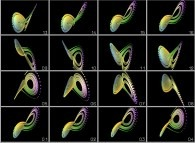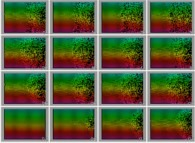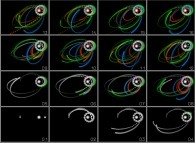# Is a Computer a Perfect Computing Machine?

## www.lactamme.polytechnique.fr

#### jean-francois.colonna@polytechnique.edu CMAP (Centre de Mathématiques APpliquées) UMR CNRS 7641, Ecole Polytechnique, CNRS, 91128 Palaiseau Cedex, France

[Site Map, Help and Search [Plan du Site, Aide et Recherche]]
[The Y2K Bug [Le bug de l'an 2000]]
[Please, visit A Virtual Machine for Exploring Space-Time and Beyond, the place where you can find thousands of pictures and animations between Art and Science]
(CMAP28 WWW site: this page was created on 02/13/2010 and last updated on 11/06/2021 10:37:43 -CET-)

[en français/in french]

Abstract: A computer is a programmable computing machine that is both finite and non continuous. Then most numbers and in particular the real numbers cannot be memorized and manipulated exactly. In most computations rounding-off errors will appear mostly in problems sensitive to initial conditions and these errors will propagate and amplify. The usual mathematical properties like associativity are lost and then two different computers regarding hardware and/or software running the same program could give different results.

Keywords: Nombres réels, Nombres Flottants, Erreurs d'arrondi, Associativité, Ordinateur, Real Numbers, Floating point numbers, Rounding-off errors, Associativity, Computer..

• Computers are everywhere and when watching their achievements, for example, with video games, one forget too often that they only "know" how to do the four elementary aritmetics operations (addition, soustraction, multiplication and division). Due to their omnipresence, one has to study the quality of their basic results.

• All numerical values we are using are written using the base 10. Let's recall that, for example, '5039.28' means:
```                        3       2       1       0       -1       -2
5x10  + 0x10  + 3x10  + 9x10  + 2x10   + 8x10
```
Unfortunately the base 10 cannot be used inside a computer for physical systems with ten stable states would be needed when they are very difficult to build. Fortunately physical systems with two stable states are everywhere: for example, an electric switch that is off or on. Then it is the base 2 that will be used.

To input a decimal number (base 10) inside a computer, a binary translation (base 2) will be requested and conversely to output a value. Inside a computer there are two main kinds of numbers:

• Integer numbers {...,-2,-1,0,+1,+2,...}.
• Floating point numbers that, in order to simplify, translate the absolute value of any number inside [1,10[ by means of a multiplication by an appropriate power of 10. For example: -5039.28=-5.03928x10^3 Then a floating point number will be defined with a small decimal number and an exponent (-5.03928 and +3 respectively in the preceding example).

• Computer memories will contain binary digits 0 and 1 (or bits). Their capacity can be huge (for example thousand billions of bits) but obviously always finite (whatever bases used!).

Most of the time, integer numbers are defined using 32 bits: one bit for the sign (0 and 1 meaning respectively '+' and '-') and 31 bits for the absolute value. Thus only the numbers between -2147483648 and +2147483647 can be defined inside a computer. The binary representation of integer numbers is precise; for example 25 is written with 32 bits:
```
00000000000000000000000000011001
```
for:
```                                         4      3      2      1      0
25 = 16 + 8 + 1 = 1x2  + 1x2  + 0x2  + 0x2  + 1x2  = 11001
```

Regarding the floating point numbers, they are using 64 bits (a 32 bit representation is available but ignored here for the sake of simplicity). Here again, only a finite set of floating point numbers can be used. For example, pi (on 12/2013 12.100.000.000.050 digits are known) cannot be input exactly inside a computer. The floating point representation of decimal numbers will be more complicated than the one of integer numbers and moreover, most of the time it will be approximate; for example 0.3 is written with 64 bits:
```                    1001 1001 1001 1001 1001 1001 1001 1010 0011 1111 1011 1001 1001 1001 1001 1001
```
whose value is very close to 0.1 but different!

• Let's see some unfortunate consequences of that when using floating point numbers during computations.

Mathematically speaking, floating point numbers are not numbers for the properties of associativity and distributivity are lost. Let's recall the respective meanings of these properties:
```
(AxB)xC = Ax(BxC)
(A+B)+C = A+(B+C)

Ax(B+C) = AxB+AxC
```
for any triplet of numbers 'A', 'B' and 'C'. Let's check that regarding the associativity running the two following small programs:
```
double    x;                                                                    double    x;
double    y;                                                                    double    y;
{                                                                               {
return(x+y);                                                                    return(x*y);
}                                                                               }

main()                                                                          main()
{                                                                               {
double    a=1.1;                                                                double    a=1.5;
double    b=3.7;                                                                double    b=2.3;
double    c=5.5;                                                                double    c=3.7;

double    x1,x2;                                                                double    x1,x2;

printf("(%.6f + %.6f) + %.6f = %.15f\n",a,b,c,x1);                              printf("(%.6f * %.6f) * %.6f = %.15f\n",a,b,c,x1);
printf("%.6f + (%.6f + %.6f) = %.15f\n",a,b,c,x2);                              printf("%.6f * (%.6f * %.6f) = %.15f\n",a,b,c,x2);
}                                                                               }

(1.100000 + 3.700000) + 5.500000 = 10.300000000000001                           (1.500000 * 2.300000) * 3.700000 = 12.764999999999999
1.100000 + (3.700000 + 5.500000) = 10.299999999999999                           1.500000 * (2.300000 * 3.700000) = 12.765000000000001
```
It is noteworthy that two functions are used to compute the addition and the multiplication respectively in order to force the respect of the order of the operations. The results obtained are different but after all the differences are very small. But are the things always so clean? Let's run this small program (for the sake of simplicity an iteration is not used):
```
main()
{
double    A,B,x0,x1,x2,x3,x4,x5,x6,x7;

B=4095.1;
A=B+1;

x0 = 1;
x1 = (A*x0) - B;
x2 = (A*x1) - B;
x3 = (A*x2) - B;
x4 = (A*x3) - B;
x5 = (A*x4) - B;
x6 = (A*x5) - B;
x7 = (A*x6) - B;

printf("x0 = %+.16f\n",x0);
printf("x1 = %+.16f\n",x1);
printf("x2 = %+.16f\n",x2);
printf("x3 = %+.16f\n",x3);
printf("x4 = %+.16f\n",x4);
printf("x5 = %+.16f\n",x5);
printf("x6 = %+.16f\n",x6);
printf("x7 = %+.16f\n",x7);
}
```
```
x0 =          +1.0000000000000000
x1 =          +1.0000000000004547
x2 =          +1.0000000018630999
x3 =          +1.0000076314440776
x4 =          +1.0312591580864137
x5 =        +129.0406374377594148
x6 =     +524468.2550088063580915
x7 = +2148270324.2415719032287598
```
It is noteworthy that this very simplistic program does not contain bugs, does not use approximation methods and at last its answers (=1) are known a priori (that is unique!). As a matter of fact the following property is true:
```                    A=B+1 ==> A-B=1 ==> x7=x6=x5=x4=x3=x2=x1=x0=1
```
Unfortunately this program does not give values equal to 1 (except the first one obviously). Where is the problem? In fact, A-B does not equal to 1; it is equal to 1 plus/minus epsilon (one single bit) for 4095.1 and 4096.1 cannot be defined accurately inside a computer using floating point numbers.

This program is more than interesting for on the one hand it exhibits clearly a general problem of computer accuracy. On the other hand, it gives an example of an "explosive" process with the fast expansion of a very smal error (the preceding single bit). At last, it makes use of a process known as iterative computation where values are transformed and transformed again following certain laws. It will be the case with the last example with the tridimensional coordinates of the four bodies (two stars and two planets). As a matter of fact, those coordinates will be defined a priori at the start of the computation and then transformed iteratively using the newtonian laws of classical Physics.

• These two "simplistic" experiments exhibit the fact that a computer is not a perfect computing machine. This is due to the impossibility to represent accurately all numbers we need.

Unfortunately, most of the useful computations done with computers make use of the real numbers. After the preceding experiments, it is obvious that it will be impossible to represent and to manipulate accurately real numbers inside a computer (except some very particular cases as the small integer numbers...).

At first, let's recall that a computer is made of hardware and programs -or softwares-. Generally speaking, two computers will not be strictly identical, in particular regarding the way they "understand" mathematical formula. For example, the following expression:
```                    (A+B)x(C+D)
```
could be "understood" as:
```                    (A+B)x(C+D)

Ax(C+D) + Bx(C+D)

AxC + AxD + BxC + BxD

etc...
```
that are equivalent mathematically speaking. But this is no longer true inside a computer due to the loss of the properties of associativity and distributivity.Then a single program running on several different computers could produce non identical results. Moreover, many time-dependent equationscan lost their property of reversibility.This experiment displays the computation of the trajectories of two planets orbiting a binary star. The computation is done using three different computers (the results of the first one are red coded, of the second one green coded and of the third one blue coded). The three computers are far to agree together and obviously the three of them are wrong.

• It is beyond all doubt that the capabilities of computers are infinite; it is true for the every day life as well for the most advanced scientific research. But it is mandatory to not forget that they are not infallible. So we have to know and master their limits in order to use them at their best.